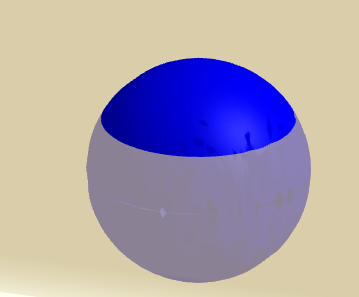# Volume and Surface Area of Spherical Cap Calculator

Two online calculators to calculate the volume, surface area and height of a sphrical cup are presented. The first calculator takes the radius of the sphere and the height of the cap and calculate the volume and area and the second calculator takes the radius of the sphere and the volume of the cap and calculate the height of the cap. The calculators also computes the ratios of the volumes and ares of the cap and the sphere from which the cap is cut.

## Spherical Cap

A spherical cap is defined as as a portion of the sphere cut by a plane.## Formulas for the Volume and Surface Area of a Spherical Cap

The volume of a spherical cap of height $h$ is given by the formula
$\displaystyle \text{Volume} = \dfrac{\pi}{3}( 3 Rh^2-h^3)$The area of the spherical cap is given by
$\displaystyle \text{Area} = 2\pi R h$
The radius $r$ of the circle whose diameter in $AB$ and the angle $\alpha$ shown in the figure above are given by
$\displaystyle \text{r} = \sqrt {R^2 - (R-h)^2 }$
$\displaystyle \alpha = \arcsin \left(\dfrac{r}{R}\right)$

## How to use the calculator

1 - Enter the radius $R$ of the sphere from which the spherical cap is cut and the height $h$ as positive real numbers, with $R \ge h$ and press "calculate". The outputs are volume $V_{cap}$ and the lateral area $A_{cap}$ of the spherical cap, the radius of the cap $r$, angle $\alpha$, the ratio $\dfrac{V_{cap}}{V_{sphere}}$ of the volume of the cap to that of the sphere and the ratio $\dfrac{A_{cap}}{A_{sphere}}$ of the area of the cap to that of the area of the shpere .

 Radius of Sphere: $R$ = 5 Height of Spherical Cap: $h$ = 1 Number of Decimals = 3

## Outputs

Enter the radius $R$ of the sphere from which the spherical cap is cut and the volume $V$ of the spherical cap as positive real numbers, with $V$ is less than half the volume of the sphee of radius $R$ and press "calculate". The outputs are the height $h$ and the lateral area $A_{cap}$ of the spherical cap, the radius of the cap $r$, angle $\alpha$, the ratio $\dfrac{V_{cap}}{V_{sphere}}$ of the volume of the cap to that of the sphere and the ratio $\dfrac{A_{cap}}{A_{sphere}}$ of the area of the cap to that of the area of the shpere .
 Radius of Sphere: $R$ = 5 Volume of Spherical Cap: $V$ = 100 Number of Decimals = 3